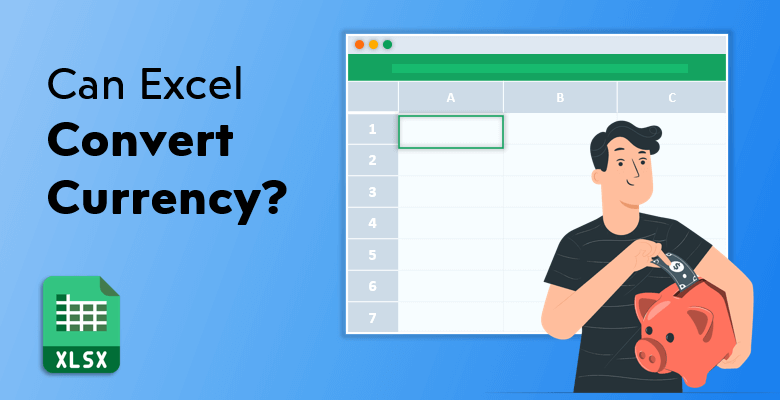# Can Excel Convert Currency?

Even if Excel does not have any built-in function to convert currency, you can still utilize some Excel functions to make it work for you.

It happens in two ways: either you provide the numeric data and Excel calculates the conversion or Excel takes the latest exchange rates from the web sources and converts currencies by using those data. Let’s look at each of them in detail.

## Convert Currency In Excel With Excel Function: Simple Currency Conversion

Basically, a currency conversion process is multiplying a certain amount by the current exchange rate. Therefore, a multiplication formula can easily be used to convert currency in Excel. Let’s say that you want to convert Euros into Dollars. Enter the amounts in Euros into a blank cell, and into the next cell enter the current exchange rate you need for conversion. Create your formula by multiplying those cells and reach the amount in Dollars. Then you can apply the formula through the range of cells.

If you have more than one type of currency to be converted, then it means things might get a little complicated. In that case, there is a particular formula in Excel that multiplies the relevant values and calculates the currency amount: VLOOKUP.

### VLOOKUP

This function is used for looking up data in a table by row. In currency conversion, it finds the wanted currencies based on the given one.

On the Excel sheet, besides the amounts to be converted, there need to be two separate tables with two columns. The to-be-calculated amount is the second column of one table, and the conversion rate is the second column of the other; both having currency types as their first column. What “VLOOKUP” does is to look in the other table for currencies and find which conversion rates they correspond to, then multiplying these rates by the given amounts.

Here is the basic formula:

=VLOOKUP (currency, xtable, column, 0) * amount

• Currency: the currency to look up
• Xtable: the currency rate table
• Column: rate field column of table
• 0: exact match
• Amount: amount to convert

You can follow these steps to convert currency by using VLOOKUP function.

1. Create your relevant data in your Excel sheet: amounts, currencies and xtable.
2. Click on the first blank under the “amount” column and enter the formula such as in the example: “VLOOKUP (D2, G2:H5, 2, 0) * B2”.
3. Apply the formula into the next cells and calculate all the wanted amounts.

## Converting with Excel Add-In: EUROTOOL.XLAM

We examined how to convert currency with simple Excel functions; now our next player is “EUROTOOL.XLAM”. This tool makes conversions through Euro; it converts Euro and other Euro member currencies using Euro as an intermediary. It uses the current exchange rates by the EU.

The syntax for Euro Currency Tool is:

=EUROCONVERT(number,source,target,full_precision,triangulation_precision).

• Number: the currency value you want to convert
• Source: a three-letter ISO code for the source currency
• Target: a three-letter ISO code for the target currency
• Full precision: it is either TRUE or FALSE. True means that all significant digits are displayed and false displays the result with the currency-specific rounding rules. False is the default one which means if you don’t enter anything, it is automatically false.
• Triangulation precision: a number equal to or greater than 3 that is used for the intermediate euro value when converting between two national currencies.

Just like a usual add-in, you first need to be sure that you installed EUROTOOL.XLAM to use. Then you can locate it into the Formulas tab.

For installing and using EUROTOOL.XLAM, follow the steps below.

1. Go to File tab, select Options then select Add-ins.
2. Make sure in the Manage list box, Excel Add-ins is selected.
3. From the Add-ins list, click “Euro Currency Tools” then click “Ok” and your tool will be installed.
4. After installing the tool, go to the Formulas tab, click Insert Function, select EUROCONVERT from the functions list and click OK.
5. Once you click OK, the Function Argument window will be opened. Provide the necessary arguments here (number, source, target etc.).
6. Your currency values will be converted.

## How to Scrap The Latest Currency Exchange Rates:

Perhaps the most exhausting part of the converting currency process is inserting the exchange rates into your sheet. Entering them one by one costs you time and effort, plus it is not really possible to have the recent rates since they are constantly changing. That’s why, if you use an external data source to take exchange rates synchronously; you wouldn’t have to worry about renewing the rates or even inserting them. Excel does it for you as long as you set up that feature.

What you need first is an online XML currency exchange rate source to import into your Excel sheet. You can use FloatRates or similar ones. Then Excel will get the latest rates and update them periodically. Make sure you have an internet connection to make it happen.

So here are the instructions to get the latest currency exchange rates:

2. Go to Data > Get External Data > From Other Sources > From XML Data Import
(In the latest versions it could be Data > Get Data > From File > From XML)
3. Copy the URL of the data source you use (FloatRates etc.) and paste it into the File name box in the Import Data window.
4. Excel will ask where do you want to put the data and you ideally choose “new worksheet”. You can also check the properties part in the same window and arrange the “name” or “refresh control” options, before clicking OK.
5. If you want to update them manually, just click Refresh All on the tab above.

You now have the current exchange rates in your Excel sheet; so you can make conversions with a simple multiplication formula or VLOOKUP function.